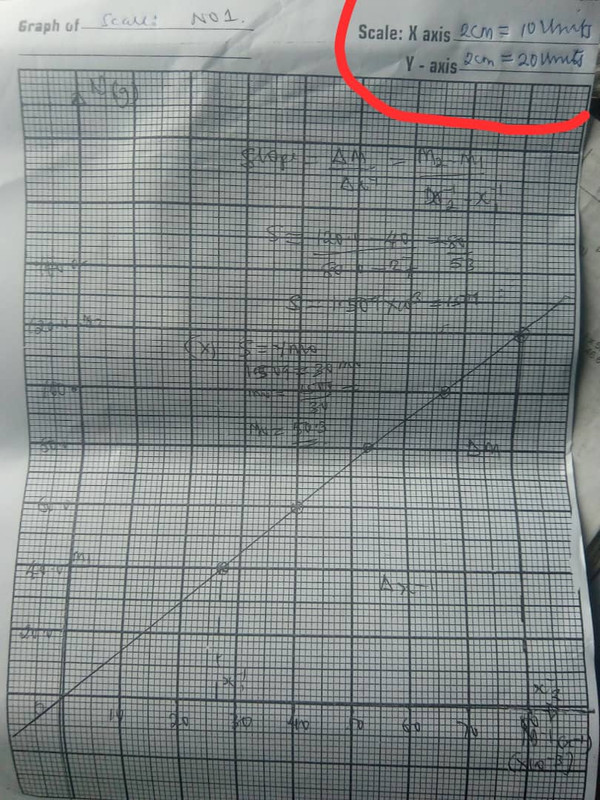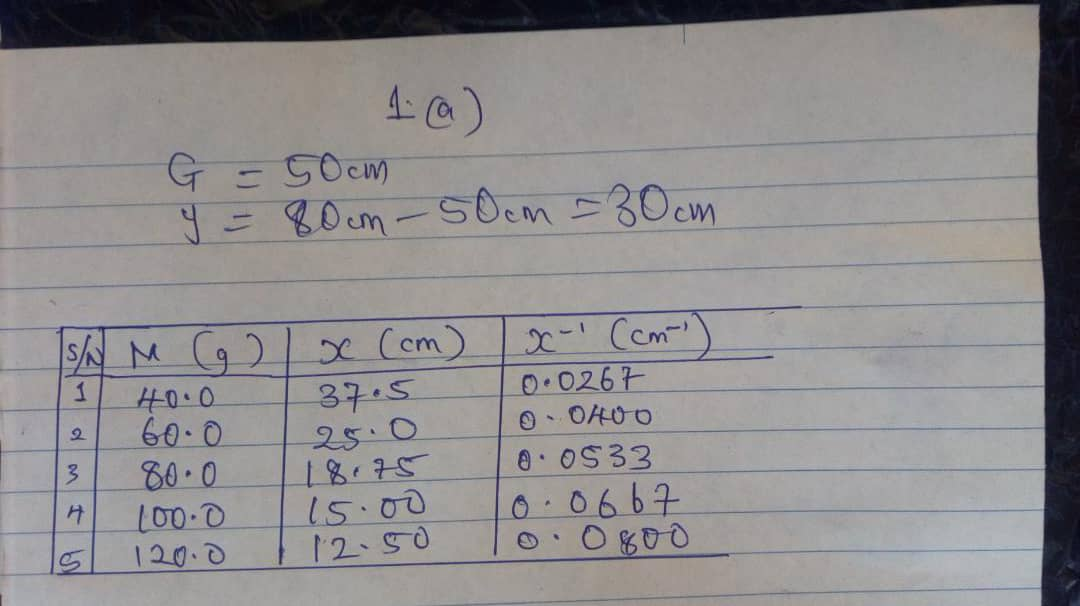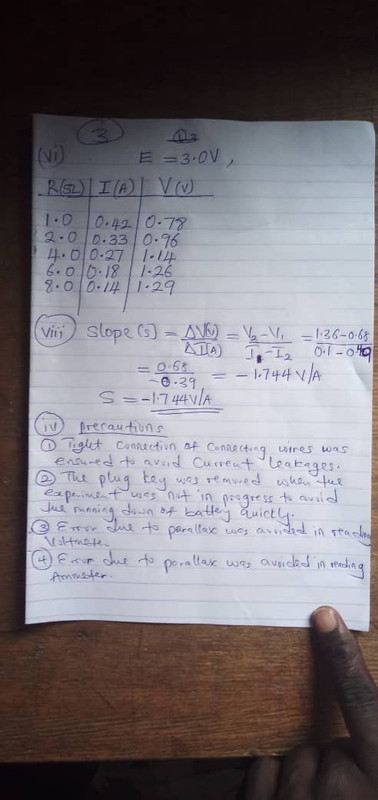# Physics Practical Waec Expo 2020 Free Answers

By 09:38 Wed, 19 Aug 2020 CommentsWaec Physics Practical Expo 2020, Waec Physics Practical Runs 2020, Physics Practical Runz 2020, Waec Physics Practical theory and Essay Answers 2020/2021, Waec Free Physics Practical free Expo 2020, Waec Physics Practical WhatsApp Group Link 2020, August 18th Physics Practical free Answers 2020

=======================

Thursday, 20th August, 2020*

Physics 3 (Practical) (Alternative A) – 08:30 hrs. – 11:15 hrs. (1st Set)

Physics 3 (Practical) (Alternative A) – 11:40 hrs. – 14:25 hrs.(2nd Set)

=======================

2020 Waec Phy Practical solution(1)

TABULATE:

S/N: 1,2,3,4,5

M(g):40.0,60.0,80.0,100.0,120.0

x(cm):37.50,25.0,18.75,15.00,12.50

x^-1(cm^-1):0.027,0.40,0.053,0.067,0.08

x^-1(*10^-3):27.00,40.00,53.00,67.00,80.00

Note: G=50.0cm Y=30.0cm

(1a)

(xi) PRECAUTIONS

(i) I ensured that the meter rule balances horizontally before readings are taken in order to avoid experimental error.

(ii) I ensured that parallax error was avoided when taking the readings on The meter rule by viewing its calibration vertically.

(1bi) Moment of a force can be defined as the product of the force and its perpendicular distance from its point of action.

Moment of a force = force * Perpendicular distance of its line of action from the point

Moment = F * L

The S.I unit is Newton-meter(Nm).

It is a vector quantity

(1bii)

x=50-25

=25cm.

y=80-50

=30cm.

From the principle of moment:

Sum of clockwise moment = Sum of anti-clockwise moment

60 * x=m * y

60 * 25 = m * 30

Divide both sides by 30

60 * 25/30 = m *30/30

m=60 * 25/30

m=2 * 25

m=50g.

++++++++++++++++++++++++++++++++++

(2)

++++++++++++++++++++++++++++++++++

(3a)

(i) E=3.00v

TABULATE.

R(ohms) | I(A) | V(v)

1.0 0.40 0.65

2.0 0.35 0.58

4.0 0.28 0.50

6.0 0.22 0.45

8.0 0.18 0.40

(3aix)

(i) I avoided error due to parallax when reading the ammeter and voltmeter.

(ii) I ensured tight connections.

(3bi)

(Pick any 2)

(i) connecting cells in parallel adds the current capacities together.

(ii) connecting cells in parallel provides alternative Pathways for Current flows.

(iii) When cells are connected in parallel the Total voltage provided do not change

(3bii)

(Pick any 2)

(i) Resistance.

(ii) Tolerance.

(iii) Rated Power Dissipation.

(iv) Package and mounting.

(iv) Voltage rating.ANSWERS LOADING...Related Article

## » WAEC 2014 PHYSICS PRACTICAL SPECIMEN ANSWERS


Comment
Name

.....................